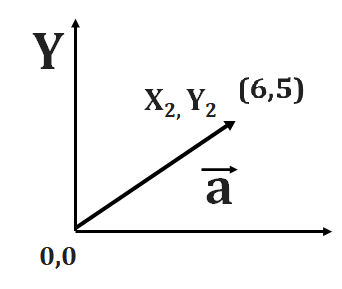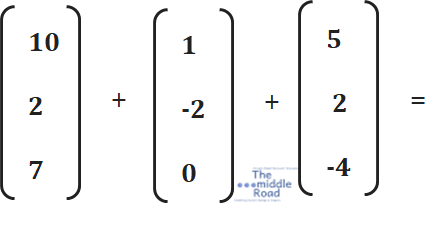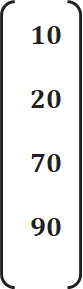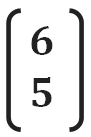Nishant Malhotra Founder of Middle Road OPC Pvt Ltd & The middle Road platform talks about the startup

### Ahoy Ahoy-Ahoy!

Welcome to the Kickass platform enabling social change & impact

# Linear Algebra problems and solutions

You are here:

### Refer to module 1 for a recap of the Linear Algebra Introduction here.

Many of the questions have solved solutions.

Q1. Do the three planes X1 + 2X+ X3  =   4,  X –  X =  1,  and X1 + 3X2  =  0 have at least one common point of intersection ? Explain

(Linear Algebra and its Application, David Lay)

X1 + 2X+ X3  =   4,

X –  X =  1,

X1 + 3X2  =   0

Ans: Recall from the lessons that systems that have at least one solution are consistent. This means they  they can either have one solution or infinite solutions. First, the system of equations is converted into augmented matrix.In the first operation, add R2 to R1 for a new R1 (R1= Row 1). For the new matrix subtract R3 from R1 TO get a new R1. You can see the new R1 has zeros and a constant 5. This leads to an equation 0X1 + 0X+0 X3  =   5there is an inherent contradiction within this equation as all the coefficients of the equation are zero and the constant or the solution of the equation is 5. There is no equation that can satisfy the rule. We are working on the triangular form and there is an inherent contradiction. This system of equations has no solution as there are no values for X1, X2,  X3  that can satisfy the equation 0 = 5.   Refer to the figure 1.

###### FIG 1 | THE MIDDLE ROAD

Q2. Do the three lines X1 – 4X = 1,  2X1  –  X =  -3,  and -X1 – 3X2  = 4  have at least one common point of intersection ? Explain

(Linear Algebra and its Application, David Lay)Q3. Find the equation involving g, h and k that makes the augmented matrix correspond to a consistent system. (Linear Algebra and its Application, David Lay) Refer to the side fig

For the solution watch the video.

Q4. In a matrix you can change the rows but not the columns

• True
• False

Q5.This augmented matrix is of the form __ * ___  ======> m*n. Define m and n.

Q6.  For a consistent system with infinite solutions, two lines _________ each other

Q7. Alice is running at a speed of 8 kms per hour. This is a _________ quantity.  Now you come to know that Alice is running in the north east direction at speed of 8 km per hour. Her speed and direction can be represent as a ______

Q8. (Maxwell’s four equations) on electromagnetic field are in which _____________________ form.

Q9. There are three column vectors. Column vectors 1 column with n rows. In this case for all column vectors n=4. Is it correct to say that we  can add all the three column vectors ?Q10. Look at the multiplication by scalars. Left the scalar is 10 and you have a column vector with coordinates in four dimensions. The new vector is equal = ?

Q11. The entropy in our universe is scalar or vector ?Q12. Look at the graph. Depict the coordinates in the X Y plane as a vector.

###### GRAPH | THE MIDDLE ROAD

Q13. In an X-Y plane, two lines are parallel. Do these lines have a consistent solution?Q14. Alice is walking to her house. She travels 3 km east, then 5 km North, and finally turns west 2 km to reach her house. Depict Alice’s journey in Vector Form.

• 2.  Yes
• 3.  2g +k + h = 0
• 4. True
• 4* 5  m= rows n = columns
• 6   Overlap
• 7. Scalar, Vector
• 8. Vector
• 9. Yes
• 10• 11 Scalar
• 12• 13 Inconsistent — No solution
• 14• 15 v= 3i+ 5j+ 2k (3, 5, 2 are scalar; speed is a scalar quantity but velocity is vector)